Next: Correct procedure Up: Constraining the mass of Previous: Analysis of experiment   Contents

## Naïve procedure

We have learned that we can infer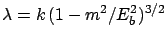and, from the relation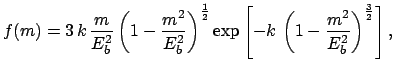we get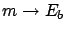(9.17)

which is clearly wrong, since it goes to 0 for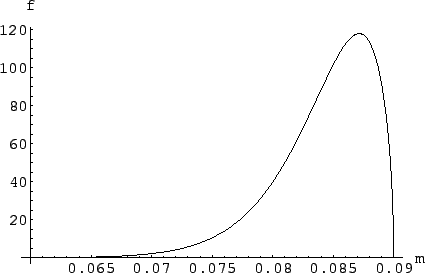. Figureshows the plot of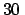, obtained with the following Mathematica code:
(********************************************************)
ClearAll["Global*"]

(* Experiment A has been run at beam energy 0.09, with
sensitivity factor k=20, threshold function beta^3,
and has observed 0 events.*)

ka=20
eba=0.09

v=Sqrt[1-(m/eba)^2]
lambda= ka*v^3

(* f(m) obtained from f(lambda)=Exp[-lambda] by lambda=k beta^3
(threshold factor) using p.d.f transformation *)

fl=Exp[-lambda]
J=Abs[D[lambda, m]]
fm=fl*J

(* check normalization and plot *)

NIntegrate[fm, {m, 0, eba}]
Plot[fm, {m, 0.06, eba}, AxesLabel -> {m, f}]

(* Strange result: try to figure out the reason! *)
(********************************************************)
`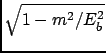The result is against our initial rational belief and we refuse to accept it. The origin of this strange behaviour is due to the term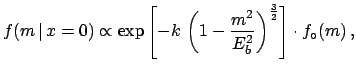in () that comes directly from the Jacobian of the transformation and, indirectly from having assumed a prior uniform in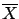. To solve the problem we have to change prior. But this seems to be cheating. Should not the prior come before? How can we change it after we have seen the final distribution?

We should not confuse what we think with how we model it. The intuitive prior is on the mass values, and this prior should be flat (at least at this stage of the analysis, as discussed in Section). Unfortunately, what is flat inis not flat in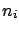, and vice versa. This problem has been discussed in Section. In fact, it is not really a problem of probability, but of extrapolating intuitive probability (which is at the basis of subjective probability and only deals with discrete numbers) to continuous variables. This is the price we pay for using all the mathematical tools of differential calculus. But one has to be very careful in formulating the problem. If one wants to get rid of these problems, one may discretizeandin a way which is consistent with to the experimental resolution. If we discretize, a flat distribution inis mapped to a flat distribution in. And the problems caused by the Jacobian go away with the Jacobian itself, at the expense of some complications in computation.Next: Correct procedure Up: Constraining the mass of Previous: Analysis of experiment   Contents
Giulio D'Agostini 2003-05-15